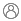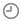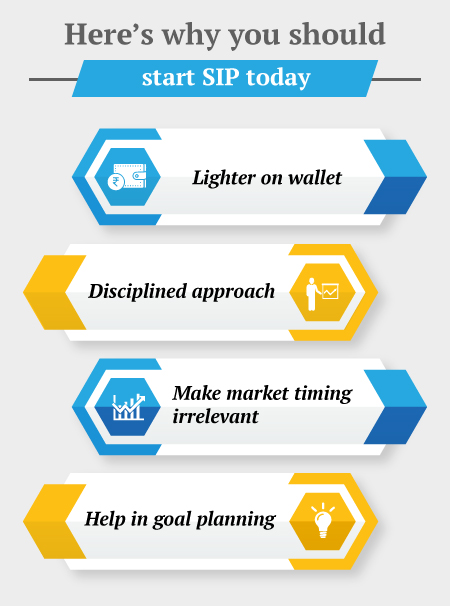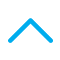To Buy: 1800-258-5899 (9 am to 6 pm)

|customerservice@canarahsbclife.in

|Locate Branch

# How to Calculate SIP Returns?Knowledge Centre TeamSeptember 23, 2021294 Views

SIP investments are made on a set date and at regular intervals. When you invest, you will be given a specific number of units based on the scheme's current NAV. You would amass a large number of units over a long period. As a result, determining your overall returns over some time can be tricky.

Returns on SIPs are determined by how much risk an investor is willing to assume. More the risk higher the reward. Because the risk is moderate in this scenario, the reward is similarly moderate. These funds can be purchased as a lump sum or as part of a Systematic Investment Plan (SIP).## Four Ways to Calculate SIP Returns

### 1. Simple Annualised Return

It's also known as effective average output, and it's a way of extending profits without presenting the full picture. Whenever the holding period is less than 12 months, a handful of investors will desire to annualize the return.

For example, in the next 8 months, or 240 days, the NAV of Rs. 20 could rise to Rs. 25. (25-20)/20 = 0.25 can be used to compute absolute returns.

We use this procedure to calculate the simple annualised returns:

(365/240) - 1 = 40.4 percent (1 + 0.25)

### 2. Absolute Return or Point-to-Point

When your investment is held for less than a year, this strategy is commonly used. The original and current or ending Net Asset Value (NAV) of the fund is all that is required. It assists you in determining the straightforward returns on your initial investment.

The term "absolute returns" refers to a fund's overall performance throughout time. It calculates the proportional increase or decrease in the NAV's value over a given period of time.

Absolute return is calculated as (current NAV – initial NAV) / initial NAV times 100.

If the present NAV is 15 and the prior NAV was 13.5, the current NAV is 15 and the prior NAV was 13.5.

Over the time period, the return would be (15 – 13.5) x 100/13.5 = 150/13.5 = 11.11 percent.

If the time period is measured in months, such as 3 months, years, such as 2, or days, such as 100, the following formula can be applied.The preceding example will yield returns of 11.11 x 4 = 44.44 percent over three months, 5.55 percent over two years, and 40.55 percent over a 100-day period.

### 3. Compound Annual Growth Rate (CAGR)

The compound annual growth rate (CAGR) is a formula that calculates the annual growth rate of an investment using the compounding effect.

CAGR is a superior approach to compute returns when the period is longer than a year. It's essentially a figure that depicts how the investment would have developed if it had a steady rate of return.

Though, returns may vary from year to year. As a result, CAGR depicts a mean annual growth rate that smooths out the variability in value over time.

Assume that an investor put Rs 1 lakh into a mutual fund three years ago at a NAV of Rs 15. The current NAV is Rs 30.

=(((ending-value/beginning-value)(1/number-of-years))-1*100 is the formula.

As a result, = (((30/15)(1/3))-1)*100 =25.99%

Use this equation if the holding time is in months: =(((ending-value/beginning-value)(12/number-of-months)) (((30/15)(12/36)-1)*100 =25.99 percent -1*100

Use Formula: =(((ending-value/beginning-value)(365/number-of-days)) if you know the NAV and the days.

-1*100 = (((30/15)(365/1095)-1)*100 = 25.99 %

### 4. XIRR Method

Income and expenses will not always be finely distributed and may occur at unpredictable times instead. XIRR is an Excel function that calculates the internal rate of return or an annualised return of a schedule of cash flows with inopportune moments.

XIRR is an Excel function that calculates the Internal Rate of Returns for a set of cash flows that occur at irregular intervals. To calculate XIRR, you'll need the following information.

a) Amount of SIP
b) SIP investments' start and end dates
c) Maturity (Redemption) Amount
d) Redemption Date

The IRR (Internal Rate of Return) takes into account the time supply of currency for investments made over time. An investor will receive a particular number of units based on the fund's NAV on that day. As a result, he should begin collecting credits the day his SIP begins.

## Why are Absolute Returns Deceptive in SIPs?

The aggregate of returns over various periods is known as point-to-point returns or absolute returns. These returns merely indicate the earnings between two dates. The earnings at a given period are displayed as point-to-point returns, which do not reflect the funds' long-term profitability.

Point-to-point returns have two limitations that may cause them to be inaccurate:

1. Returns are for a specific period of time.
2. The events of the time are not taken into account.

Because the capital flows into the investment portfolio are delayed and not deposited all at once, the absolute return number has limited validity in the case of SIPs. In other words, if an investor had made a one-time investment and gained a profit over a year, his estimate of the return would've been absolutely right.

One method to get around the limits of point-to-point returns is to look at the performance of the funds over a longer period of time. A longer period takes into account the many market cycles, resulting in a more realistic picture.

The returns on these SIPs do not have to be calculated by the investor. More than anything, what matters to an investor is that they understand how SIP returns are calculated.

After going over the procedures for computing SIP returns for mutual funds, an investor will be able to calculate SIP returns correctly. Furthermore, formulas from various categories have been thoroughly described so that an investor can make the best decision possible when investing in SIP and estimate returns appropriately.

### Browse by Categories##### Try OurCalculators### Get a Call Back

Do you want us to call back Please fill the form belowAnnual Income (In Lacs)### Our ProductsiSelect Smart360 Term Plan

Life Cover till 99 years of age

Option to Block the premium rate and increase cover by upto 100% at the blocked rate

Option to avail monthly income post attaining 60 years of age

Tax Benefits as per applicable lawsGuaranteed Savings Plan

Better value for high premium commitment

Guaranteed benefits payable on maturity

Life cover for the entire term

Flexibility to choose premium payment termsGuaranteed Income4Life Plan

Guaranteed lifelong income up to 99 years of ageCall BackPay Premium